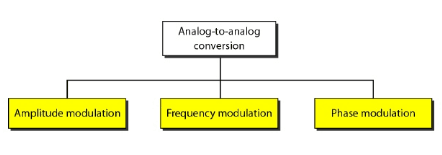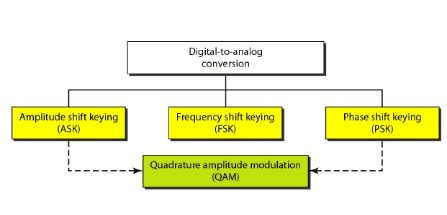Digital-to-analog conversion is the process of changing one of the characteristics of an analog signal based on the information in the digital data.

Digital-to-analog conversion can be accomplished in several ways: amplitude shift keying (ASK), frequency shift keying (FSK), and phase shift keying (PSK). Quadrature amplitude modulation (QAM) combines ASK and PSK.

In amplitude shift keying, the amplitude of the carrier signal is varied to create signal elements. Both frequency and phase remain constant while the amplitude changes.

In frequency shift keying, the frequency of the carrier signal is varied to represent data. The frequency of the modulated signal is constant for the duration of one signal element, but changes for the next signal element if the data element changes. Both peak amplitude and phase remain constant for all signal elements.

In phase shift keying, the phase of the carrier is varied to represent two or more different signal elements. Both peak amplitude and frequency remain constant as the phase changes.

A constellation diagram shows us the amplitude and phase of a signal element, particularly when we are using two carriers (one in-phase and one quadrature).

Quadrature amplitude modulation (QAM) is a combination of ASK and PSK. QAM uses two carriers, one in-phase and the other quadrature, with different amplitude levels for each carrier.

Analog-to-analog conversion is the representation of analog information by an analog signal. Conversion is needed if the medium is bandpass in nature or if only a bandpass bandwidth is available to us.

Analog-to-analog conversion can be accomplished in three ways: amplitude modulation (AM), frequency modulation (FM), and phase modulation (PM).

In AM transmission, the carrier signal is modulated so that its amplitude varies with the changing amplitudes of the modulating signal. The frequency and phase of the carrier remain the same; only the amplitude changes to follow variations in the information.

In PM transmission, the frequency of the carrier signal is modulated to follow the changing voltage level (amplitude) of the modulating signal. The peak amplitude  and phase of the carrier signal remain constant, but as the amplitude of the information signal changes, the frequency of the carrier changes correspondingly.

In PM transmission, the phase of the carrier signal is modulated to follow the
changing voltage level (amplitude) of the modulating signal. The peak amplitude
and frequency of the carrier signal remain constant, but as the amplitude of the
information signal changes, the phase of the carrier changes correspondingly.